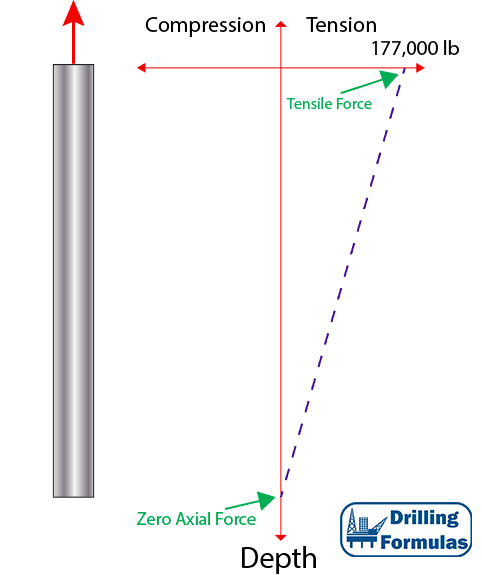## Buoyancy Effect on Weight of Tubular Submersed in FluidWhen a tubular submerses into drilling fluid or completion fluid, it will affect how the force distribution works on the tubular. This article will describe how buoyancy will affect tubular weight and a location of neutral axial load (zero axial load).

Weight of Tubing in Air

When tubing is hung in the air, the string weight is equal to linear weight per foot multiplied by the total length of the string. The maximum tensile load is on surface and the zero axial load point is at the bottom.

Tubing Detail

• 5” Tubing
• Weight per length = 17.7 lb/ft
• Total Length = 10,000 ft

Total weight = 17.7 x 10,000 = 177,000 lb

Weight at the bottom of tubing is 0 and weight on top is 177,000 lb in tensile.

Figure 1 demonstrates force distribution of tubing hanging in air.Figure 1 – Weight of Tubing in Air

Weight of Tubing When Submersed in Fluid Continue reading